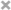# mysql存储过程

1、存储过程的概念

2、创建存储过程

```create procedure procedureName()
begin
//--sql 语句
end\$```

3、查看已有的存储过程

`show procedure status`

4、删除存储过程

`drop procedure procedureName;`

5、调用存储过程

`call procedureName();`

6、第一个存储过程

```create procedure p1()
begin
select 2+3;
end\$```

`call p1();`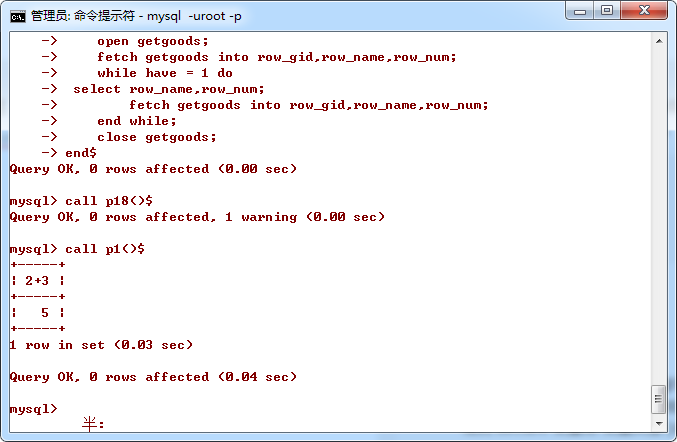7、引入变量

declare 变量名 变量类型 [default 默认值]

```create procedure p2()
begin
declare age int default 18;
declare height int default 180;
select concat('年龄：',age,'身高：',height);
end\$```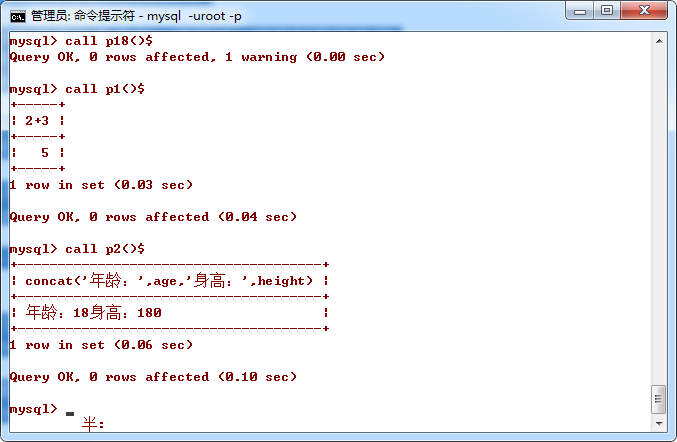8、引入表达式

`set 变量名:= expression`

```create procedure p3()
begin
declare age int default 18;
set age := age + 20;
select concat('20年后年龄：',age);
end\$```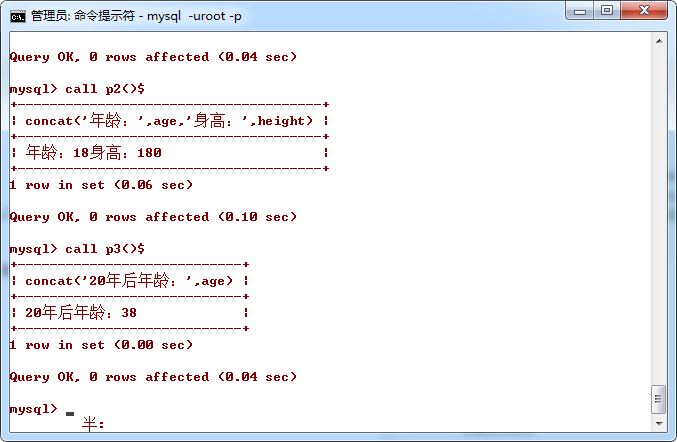9、引入选择控制结构

```if condition then
statement
elseif
statement
else
statement
end if;```

```create procedure p4()
begin
declare age int default 18;
if age >= 18 then
select '已成年';
else
select '未成年';
end if;
end\$```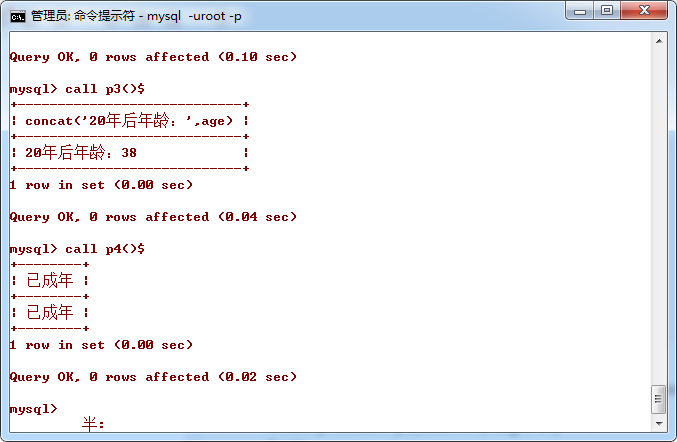10、给存储过程传参

`[in/out/inout] 参数名 参数类型`

```create procedure p5(width int,height int)
begin
select concat('你的面积是：',width * height) as area;
if width > height then
select '你比较胖';
elseif width < height then
select '你比较瘦';
else
select '你比较方';
end if;
end\$```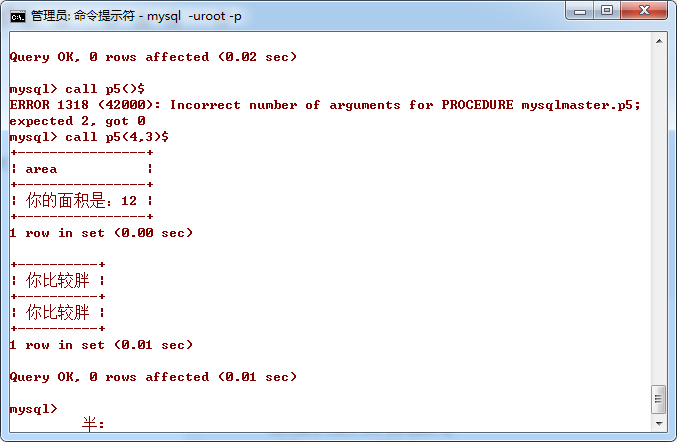11、使用while循环结构

```create procedure p6()
begin
declare total int default 0;
declare num int default 0;
while num <= 100 do
set total := total + num;
set num := num + 1;
end while;
select total;
end\$```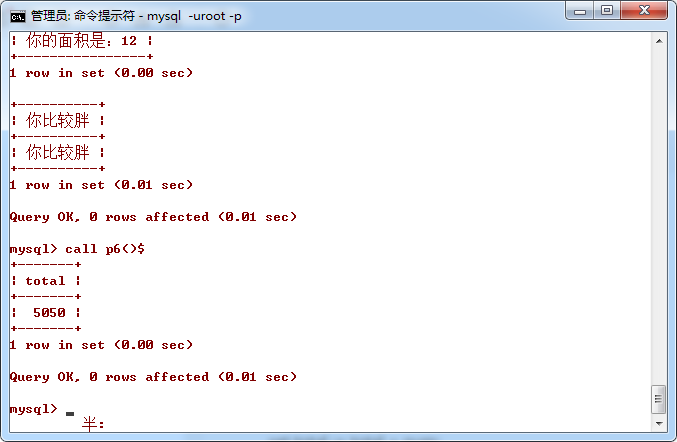12、存储过程参数的输入输出类型

（1）in型，此时in为输入行参数，它能接受到我们的输入

```create procedure p7(in n int)
begin
declare total int default 0;
declare num int default 0;
while num <= n do
set total := total + num;
set num := num + 1;
end while;
select total;
end\$```

`call p7(100);`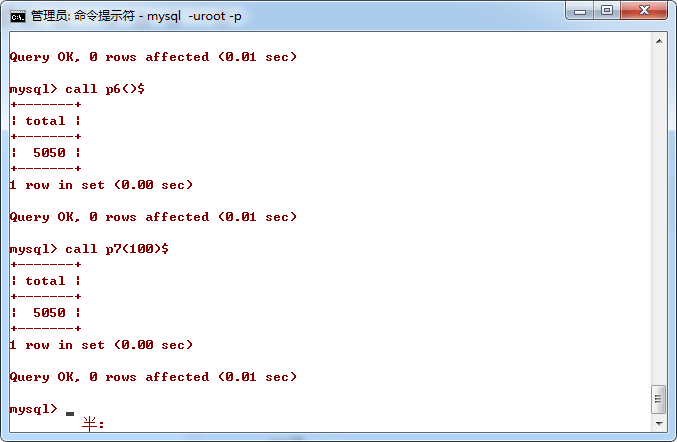（2）out类型的参数

```create procedure p8(in n int,out total int)
begin
declare num int default 0;
set total := 0;
while num <= n do
set total := total + num;
set num := num + 1;
end while;
end\$```

```call p8(100,@total); --100为输入型参数，而@total为输出型变量
select @total; --输出@total变量```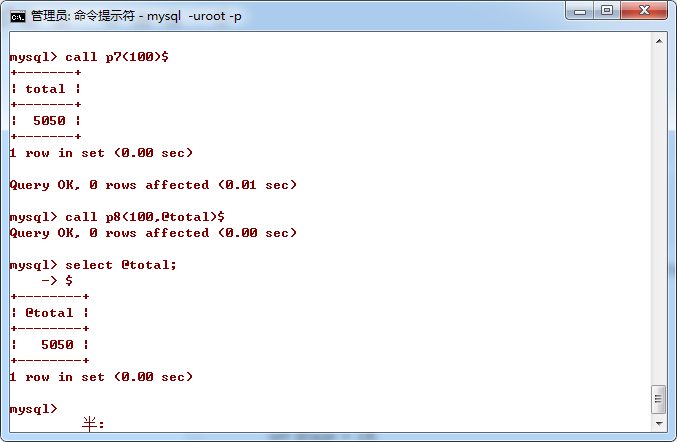（3）inout类型的参数

```create procedure p9(inout age int)
begin
set age := age+20;
end\$```

```set @age = 18; --设置@age变量为18
call p9(@age); --调用p9存储过程，@age变量为实参
select @age; --显示@age变量```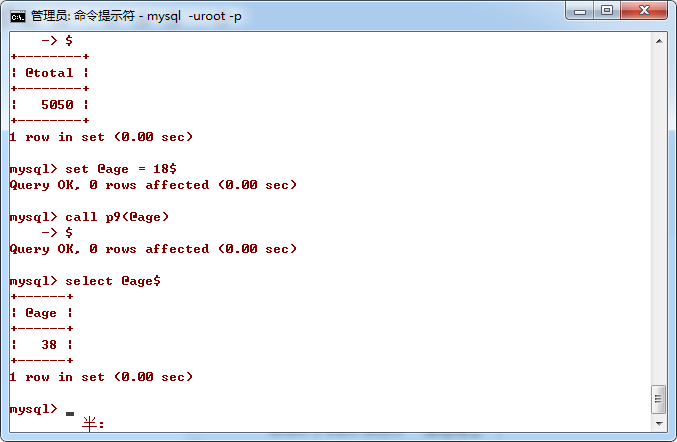inout型变量的实参也是一个变量名，这个变量在存储过程中既作为输入变量，又作为输出变量。

13、case结构的用法

```create procedure p10()
begin
declare pos int default 0;
set pos := floor(5*rand());
case pos
when 1 then select '仍然在飞';
when 2 then select '落在海里';
when 3 then select '落在陆上';
else select '我不知道在哪里';
end case;
end\$```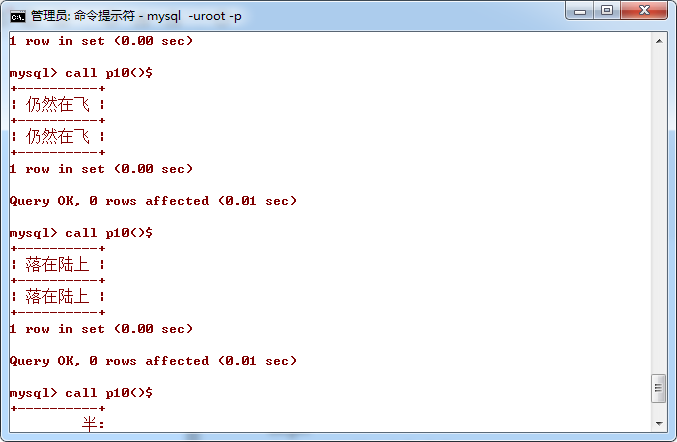14、repeat循环结构

```[begin_label:] REPEAT
statement_list
UNTIL search_condition
END REPEAT [end_label]```

```create procedure p11()
begin
declare total int default 0;
declare num int default 0;
r:repeat
set total:= total + num;
set num:=num + 1;
until num > 100
end repeat r;
select total;
end\$```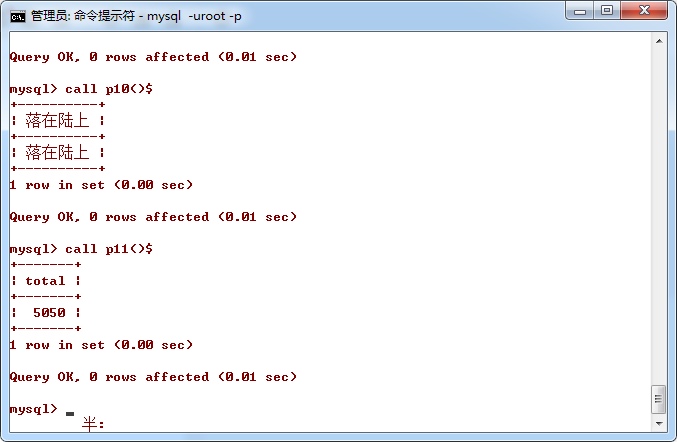https://www.jb51.net/article/164677.htm• 最新评论
• 总共条评论